# Boomerang Attack in Cryptography

Cryptography | Boomerang Attack: In this tutorial, we will briefly study the basis of boomerang attack and examples aim to capture. We will cover types of messages in Boomerang Attack. By Monika Sharma Last updated : May 25, 2023

## What is Boomerang Attack in Cryptography?

Here, in cryptography, the boomerang attack is a method for the cryptanalysis of block ciphers based on differential cryptanalysis in cryptography. Thus, the attack was published in 1999 by David Wagner who used it to break the COCONUT98 cipher in cryptography as a block cipher.

Hence, the boomerang attack has allowed new avenues of attack for many ciphers previously deemed safe from differential cryptanalysis in cryptography.

This attack has several interesting applications in this. Hence, mainly in starting, we don't have to prove the t-repeated claim that eliminating all high-probability differentials for the whole cipher is sufficient to guarantee security against differential attacks in this algorithm in cryptography. Then further is as, where we show how to break COCONUT98, a cipher designed using decorrelation techniques to ensure provable security against differential attacks in this, with an advanced differential-style attack that needs just 216 adaptively chosen texts as an original text or a plain text. Then, also to illustrate the power of boomerang techniques, we give new attacks on Khufu-16, FEAL-6, and 16 rounds of CAST-256 and furthermore.

When the boomerang attack is based on differential cryptography in cryptoanalysis. Hence, in differential cryptanalysis, an attacker exploits how differences in the input to a cipher or the plaintext or the original text can affect the resultant difference at the output or the ciphertext in cryptography. Thus, in the way of this algorithm a high-probability "differential" that is, an input difference that will produce a likely output difference is needed that covers all, or nearly all, of the cipher in cryptography. Thus, the boomerang attack allows differentials to be used which cover only part of the cipher in the cryptography.

Whenever refinements on the boomerang attack have been published or described: the amplified boomerang attack, then the rectangle attack in cryptography for these attacks.

Thus, the main idea behind the boomerang attack is to use two short differentials with high probabilities instead of one differential of more rounds with low probability in the algorithm to attacks cryptography.
Hence, the motivation for such an attack is quite apparent, as it is easier to find short differentials with a high probability than finding a long one with a high enough probability in the algorithm to attacks on cryptography.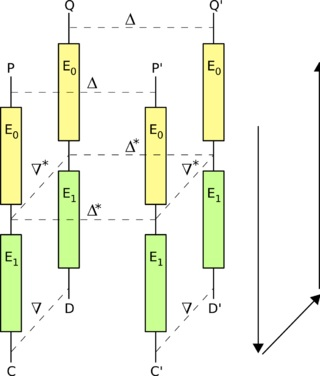## The Process of Boomerang Attack

When the boomerang attack is based on differential cryptography in cryptoanalysis. Hence, in differential cryptanalysis, an attacker exploits how differences in the input to a cipher or the plaintext or the original text can affect the resultant difference at the output or the ciphertext in cryptography. Thus, in the way of this algorithm a high-probability "differential" that is, an input difference that will produce a likely output difference is needed that covers all, or nearly all, of the cipher in cryptography. Thus, the boomerang attack allows differentials to be used which cover only part of the cipher in the cryptography.

Hence, the attack attempts to generate a so-called "quartet" structure at a point halfway through the cipher in cryptography. Hence, we say that the encryption action, E, of the cipher can be split into two consecutive stages, E0 and E1, so that E(M) = E1(E0(M)), where M is some plaintext message or the original text message. Here, we suppose we have two differentials for the two stages; we say,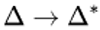Here, for E0, and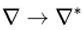or E1-1 or the decryption action of E1 in this

## The basic attack proceeds as follows

• Firstly, choose a random plaintext P and calculate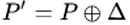• Then, request the encryptions of P and P' to obtain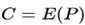and• Then, Calculate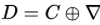and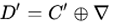• Then, request the decryptions of D and D' to obtain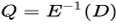and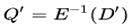• And then, compare Q and Q' when the differentials hold,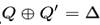Reference: Boomerang attack